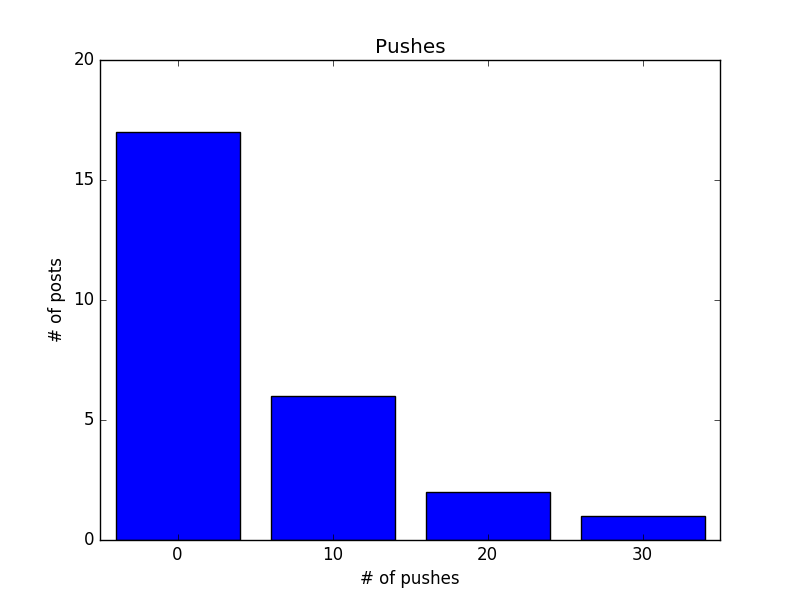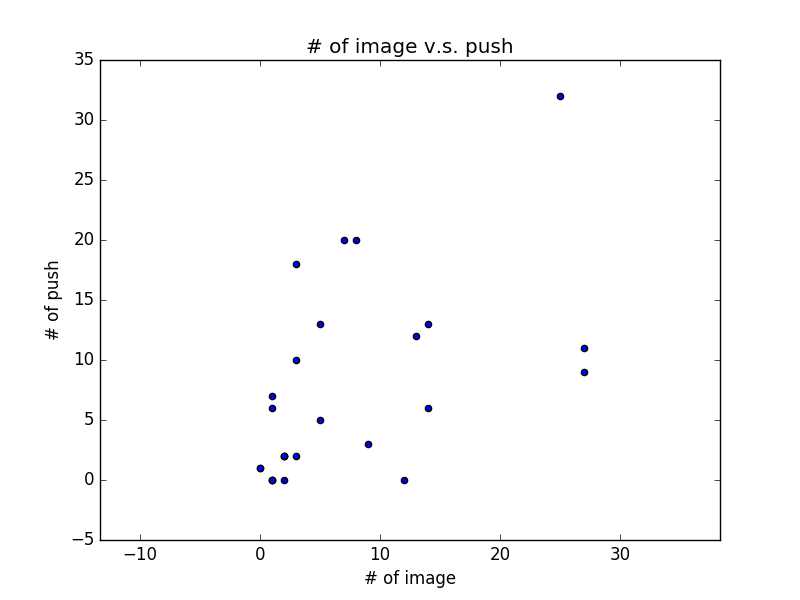with open('example.json', 'r', encoding='utf-8') as f:
images = []
pushes = []
for d in data_list:
images.append(d['num_image'])
pushes.append(d['push_count'])

print('圖片數:', images, 'Max:', max(images), 'Min:', min(images))
print('推文數:', pushes, 'Max:', max(pushes), 'Min:', min(pushes))

# 圖片數: [3, 7, 1, 12, 9, 1, 2, 13, 0, 5, 27, 5, 1, 8, 0, 1, 14, 2, 3, 2, 1, 25, 3, 14, 27, 2] Max: 27 Min: 0
# 推文數: [18, 20, 0, 0, 3, 6, 2, 12, 1, 13, 11, 5, 0, 20, 1, 7, 6, 2, 2, 0, 0, 32, 10, 13, 9, 2] Max: 32 Min: 0


## 平均值與相關係數

def mean(x):
return sum(x) / len(x)

print('平均圖片數:', mean(images), '平均推文數:', mean(pushes))

# 平均圖片數: 7.230769230769231 平均推文數: 7.5


def de_mean(x):
x_bar = mean(x)
return [x_i - x_bar for x_i in x]

def variance(x):
deviations = de_mean(x)
variance_x = 0
for d in deviations:
variance_x += d**2
variance_x /= len(x)
return variance_x

def dot(x, y):
dot_product = sum(v_i * w_i for v_i, w_i in zip(x, y))
dot_product /= (len(x))
return dot_product


def correlation(x, y):
variance_x = variance(x)
variance_y = variance(y)
sd_x = math.sqrt(variance_x)
sd_y = math.sqrt(variance_y)
dot_xy = dot(de_mean(x), de_mean(y))
return dot_xy/(sd_x*sd_y)

print('相關係數:', correlation(images, pushes))

# 相關係數: 0.5258449106844523


• x 導致 y
• y 導致 x
• x, y 互為因果
• 另有他因 z 導致 x, y (例如高 GDP 同時導致高平均壽命與高基礎網路頻寬，平均壽命與基礎網路頻寬並沒有因果關係)
• x, y 根本無關，只是巧合 (例如一個地區的手機銷售量與律師人數?)

## 資料視覺化

def decile(num):  # 將數字十分位化
return (num // 10) * 10

from collections import Counter
histogram = Counter(decile(push) for push in pushes)
print(histogram)
# Counter({0: 17, 10: 6, 20: 2, 30: 1})

# 畫出 histogram
from matplotlib import pyplot as plt

plt.bar([x-4 for x in histogram.keys()], histogram.values(), 8)
plt.axis([-5, 35, 0, 20])
plt.title('Pushes')
plt.xlabel('# of pushes')
plt.ylabel('# of posts')
plt.xticks([10 * i for i in range(4)])
plt.show()plt.scatter(images, pushes)
plt.title('# of image v.s. push')
plt.xlabel('# of image')
plt.ylabel('# of push')
plt.axis('equal')
plt.show()## 結語：資料科學的三個面向

• 資料處理，寫程式，架系統，對應資料的來源、儲存、處理、查詢等，
• 統計分析，數學，各種機器學習模型與技巧
• Domain knowledge，也就是到底要對著資料問什麼問題